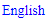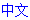# Supercoloring

## How it Works

The technique operates on all remaining candidates in the grid, excluding the givens and the solved cells. Although it considers all remaining candidates, it is still a single-digit solving technique. Interactions between different digits are not taken into consideration.

### Assign Colors

Each remaining candidate receives a different color. For practical purposes, letters are used to represent these colors. In this stage, conjugate pairs are ignored. A grid with 50 remaining candidates will have 50 different colors.

### Build Exclusion Matrix

Create a list of all color pairs which are mutually exclusive, because they represent the same digit in a single house. You only need half the matrix. A excludes B also implies B excludes A.

### Build Conjugate Matrix

Create a list of all color pairs which form a conjugate pair in one or more houses.

### Recolor Equivalent Colors

When A is conjugate to B and A is conjugate to C, B and C are equivalent. Every C is replaced by B in the results. This process is repeated until no more colors can be merged. At the end, each pair of conjugate colors represents a color cluster.

### Build Implication Matrix

Using the Exclusion Matrix and the Conjugate Matrix, a third matrix is calculated. The following rule is used:

When A excludes B and B is conjugate to C, A implies C. This matrix only works in a single direction. When A implies C, it is not true that C also implies A.

### Evaluate the Results

When A implies B and A implies C and B excludes C, we have found a contradiction, allowing us to eliminate the candidates for color A.
The Identity rule
When A implies B and B implies A, A and B are equivalent and we can replace all instances of B by A. After this recoloring, we may be able to expand the Exclusion Matrix.

## Supercoloring and other Coloring Techniques

Supercoloring did not survive as a solving technique, but its remnants can be found in Multi-Colors and other coloring techniques. Although these techniques use the same principles, the process is simplified so it can be performed by human solvers.

## Sudoku strategies

﻿## Printable Sudoku